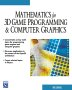# Maths - Trigonometry - Derived Trig Functions

## Double Angle Formula

Since quaternions use expressions like sin(t/2) and cos(t/2) it would be useful to have expressions for these in terms of sin(t) and cos(t)

As a starting point take the following trig functions:

sin(2A) = 2 sin(A) cos(A)

cos(2A) = 2 cos²(A) - 1 = 1 - 2 sin²(A)

where:

• cos²(A) is shorthand notation for (cos(A))², that is, the square of cos
• sin²(A) is shorthand notation for (sin(A))², that is, the square of sine

## Half Angle Formula

• sin(t/2) =√(0.5 (1- cos(t)))
• cos(t/2) =√(0.5 (1+ cos(t)))
• tan(t/2) = sin(t)/(1+cos(t))### Graphical Representation

We can show these relationships graphically
where the angle is shown at the centre of a
unit circle and the half angle is the angle at
a point on the circumference.

### Derivation

In the above double angle formula we substitute t=2A to give:

1 - 2 sin²(t/2) = cos(t)

sin²(t/2) =0.5 (1- cos(t))

sin(t/2) =√(0.5 (1- cos(t)))

Similarly for cosine:

2 cos²(t/2) - 1 = cos(t)

2 cos²(t/2) = 1 + cos(t)

cos(t/2) =√(0.5 (1+ cos(t)))

 metadata block see also: Correspondence about this page Book Shop - Further reading. Where I can, I have put links to Amazon for books that are relevant to the subject, click on the appropriate country flag to get more details of the book or to buy it from them.Mathematics for 3D game Programming - Includes introduction to Vectors, Matrices, Transforms and Trigonometry. (But no euler angles or quaternions). Also includes ray tracing and some linear & rotational physics also collision detection (but not collision response). Other Math Books

This site may have errors. Don't use for critical systems.